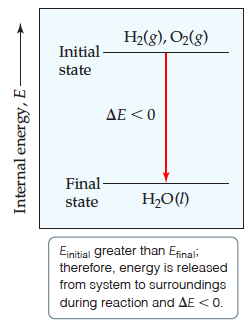# Problem: Energy diagram for the reaction 2 H2(g) + O2(g) → 2 H2O(l) is shown below.The internal energy for Mg(s) and Cl2(g) is greater than that of MgCl2(s). Sketch the energy diagram that represents the reaction MgCl2(s) → Mg(s) + Cl2(g).

###### FREE Expert Solution

We are given the energy diagram for the reaction 2 H2(g) + O2(g) → 2 H2O(l):We are then asked to sketch the energy diagram that represents the reaction MgCl2(s) → Mg(s) + Cl2(g)

95% (140 ratings)###### Problem Details

Energy diagram for the reaction 2 H2(g) + O2(g) → 2 H2O(l) is shown below.The internal energy for Mg(s) and Cl2(g) is greater than that of MgCl2(s). Sketch the energy diagram that represents the reaction MgCl2(s) → Mg(s) + Cl2(g).